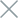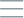• Home
• Tags
• Series
•# Dynamic Search Algorithm in C++ - Explanation, Example and Code

The dynamic search algorithm, also known as the "search-as-you-type" algorithm, is commonly used to implement features like autocomplete in search bars. This algorithm provides real-time suggestions based on the user's input and the available data.

## How It Works

1. Start with a dataset containing a list of items (e.g., words, names or products).
2. As the user types each character, update the search query.
3. Filter the dataset based on the current search query.
4. Display the filtered results to the user in real-time.

## Example

Consider a dataset of programming languages: ["C", "C++", "Java", "Python", "JavaScript", "Ruby", "Swift"].

1. User types "C". Filtered results: ["C", "C++"].
2. User types "C++". Filtered results: ["C++"].
3. User types "Java". Filtered results: ["Java", "JavaScript"].
4. User types "Py". Filtered results: ["Python"].
5. User types "Jav". Filtered results: ["Java", "JavaScript"].

## Example Code in C++

``````#include <iostream>
#include <vector>
#include <string>

std::vector<std::string> dynamicSearch(const std::vector<std::string>& dataset, const std::string& query) {
std::vector<std::string> results;

for (const std::string& item : dataset) {
if (item.find(query) != std::string::npos) {
results.push_back(item);
}
}

return results;
}

int main() {
std::vector<std::string> programmingLanguages = {"C", "C++", "Java", "Python", "JavaScript", "Ruby", "Swift"};
std::string userQuery = "Jav";

std::vector<std::string> suggestions = dynamicSearch(programmingLanguages, userQuery);

std::cout << "Suggestions for query '" << userQuery << "': ";
for (const std::string& suggestion : suggestions) {
std::cout << suggestion << " ";
}
std::cout << std::endl;

return 0;
}
``````

In this example, the `dynamicSearch` function takes a dataset of programming languages and a user query as inputs. It returns suggestions based on the current query. As the user types characters, the algorithm filters the dataset and displays real-time suggestions.

Note: The actual implementation of dynamic search can be more complex, involving techniques like trie structures or efficient indexing for large datasets.